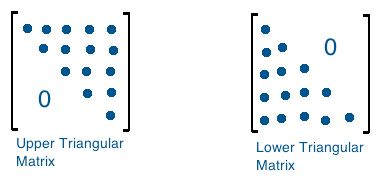# If A is a upper triangular matrix of order 3 x 3 then which of the following is TRUE?  Option 1) Option 2) Option 3) Option 4) none of these

H Himanshu

As we have learned

Upper Triangular Matrix -

A square matrix in which all the elements below the principal diagonal are Zero

- whereinFor upper triangular matrices ,   i.e element below the diagonal

Option 1)

Option 2)

Option 3)

Option 4)

none of these

Exams
Articles
Questions